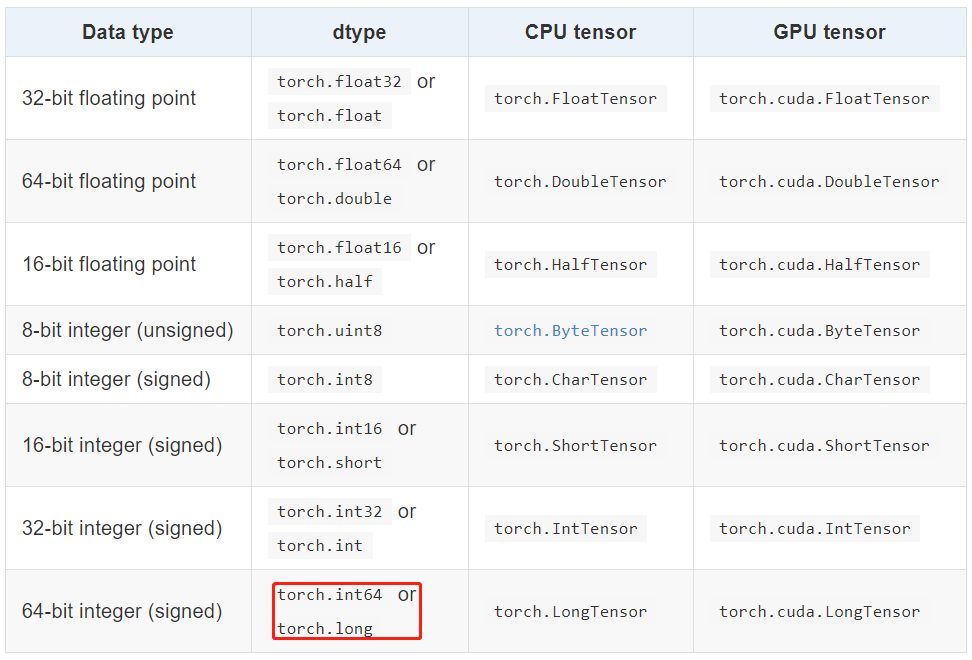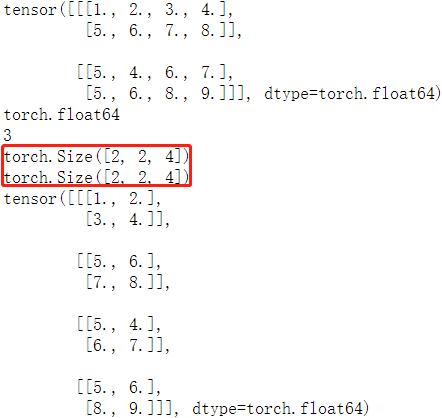# Pytorch基礎-tensor資料結構

## torch.Tensor

`torch.Tensor` 是一種包含單一資料型別元素的多維矩陣，類似於 numpy 的 `array`。 可以使用使用 torch.tensor() 方法將 python 的 list 或序列資料轉換成 Tensor 資料，生成的是`dtype` 預設是 `torch.FloatTensor`

1，指定資料型別的 tensor 可以通過傳遞引數 `torch.dtype` 和/或者 `torch.device` 到建構函式生成：

```python

torch.ones([2,3], dtype=torch.float64, device="cuda:0") tensor([[1., 1., 1.], [1., 1., 1.]], device='cuda:0', dtype=torch.float64) torch.ones([2,3], dtype=torch.float32) tensor([[1., 1., 1.], [1., 1., 1.]]) ```

2，Tensor 的內容可以通過 Python 索引或者切片訪問以及修改：

```python

matrix = torch.tensor([[2,3,4],[5,6,7]]) print(matrix) tensor(7) matrix = 9 print(matrix) tensor([[2, 3, 4], [5, 6, 9]]) ```

3，使用 `torch.Tensor.item()` 或者 `int()` 方法從只有一個值的 Tensor中獲取 Python Number：

```python

x = torch.tensor([[4.5]]) x tensor([[4.5000]]) x.item() 4.5 int(x) 4 ```

4，Tensor可以通過引數 `requires_grad=True` 建立, 這樣 `torch.autograd` 會記錄相關的運算實現自動求導：

```python

x = torch.tensor([[1., -1.], [1., 1.]], requires_grad=True) out = x.pow(2).sum() out.backward() x.grad tensor([[ 2.0000, -2.0000], [ 2.0000, 2.0000]]) ```

5，每一個 tensor都有一個相應的 `torch.Storage` 儲存其資料。tensor 類提供了一個多維的、strided 檢視, 並定義了數值操作。

## Tensor 資料型別

Torch 定義了七種 CPU Tensor 型別和八種 GPU Tensor 型別：`torch.Tensor` 是預設的 tensor 型別（`torch.FloatTensor`）的簡稱，即 `32` 位浮點數資料型別。

## Tensor 的屬性

Tensor 有很多屬性，包括資料型別、Tensor 的維度、Tensor 的尺寸。

• 資料型別：可通過改變 torch.tensor() 方法的 `dtype` 引數值，來設定不同的 `Tensor` 資料型別。
• 維度：不同型別的資料可以用不同維度(dimension)的張量來表示。標量為 `0` 維張量，向量為 `1` 維張量，矩陣為 `2` 維張量。彩色影象有 `rgb` 三個通道，可以表示為 `3` 維張量。影片還有時間維，可以表示為 `4` 維張量，有幾個中括號 `[` 維度就是幾。可使用 `dim() 方法` 獲取 `tensor` 的維度
• 尺寸：可以使用 `shape屬性`或者 `size()方法`檢視張量在每一維的長度，可以使用 `view()方法`或者`reshape() 方法`改變張量的尺寸。Pytorch 框架中四維張量形狀的定義是 `(N, C, H, W)`

```python matrix = torch.tensor([[[1,2,3,4],[5,6,7,8]], [[5,4,6,7], [5,6,8,9]]], dtype = torch.float64) print(matrix) # 列印 tensor print(matrix.dtype) # 列印 tensor 資料型別 print(matrix.dim()) # 列印 tensor 維度 print(matrix.size()) # 列印 tensor 尺寸 print(matrix.shape) # 列印 tensor 尺寸 matrix2 = matrix.view(4, 2, 2) # 改變 tensor 尺寸 print(matrix2)```### view 和 reshape 的區別

• 兩個方法都是用來改變 tensor 的 shape，view() 只適合對滿足連續性條件（`contiguous`）的 tensor 進行操作，而 reshape() 同時還可以對不滿足連續性條件的 tensor 進行操作。
• 在滿足 tensor 連續性條件（`contiguous`）時，a.reshape() 返回的結果與a.view() 相同，都不會開闢新記憶體空間；不滿足 `contiguous` 時， 直接使用 view() 方法會失敗，`reshape()` 依然有用，但是會重新開闢記憶體空間，不與之前的 tensor 共享記憶體，即返回的是 ”副本“（等價於先呼叫 `contiguous()` 方法再使用 `view()` 方法）。 更多理解參考這篇文章

## Tensor 與 ndarray

1，張量和 numpy 陣列。可以用 `.numpy()` 方法從 Tensor 得到 numpy 陣列，也可以用 `torch.from_numpy` 從 numpy 陣列得到Tensor。這兩種方法關聯的 Tensor 和 numpy 陣列是共享資料記憶體的。可以用張量的 `clone`方法拷貝張量，中斷這種關聯。

```python arr = np.random.rand(4,5) print(type(arr)) tensor1 = torch.from_numpy(arr) print(type(tensor1)) arr1 = tensor1.numpy() print(type(arr1)) """ <class 'numpy.ndarray'> <class 'torch.Tensor'> <class 'numpy.ndarray'> """```

2，`item()` 方法和 `tolist()` 方法可以將張量轉換成 Python 數值和數值列表

```python

# item方法和tolist方法可以將張量轉換成Python數值和數值列表

scalar = torch.tensor(5) # 標量 s = scalar.item() print(s) print(type(s))

tensor = torch.rand(3,2) # 矩陣 t = tensor.tolist() print(t) print(type(t)) """ 1.0 [[0.8211846351623535, 0.20020723342895508], [0.011571824550628662, 0.2906131148338318]] """ ```

## 建立 Tensor

### 傳入維度的方法

|方法名|方法功能|備註| |-----|-------|---| |`torch.rand(*sizes, out=None) → Tensor`|返回一個張量，包含了從區間 `[0, 1)`均勻分佈中抽取的一組隨機數。張量的形狀由引數sizes定義。|推薦| |`torch.randn(*sizes, out=None) → Tensor`|返回一個張量，包含了從標準正態分佈（均值為0，方差為1，即高斯白噪聲）中抽取的一組隨機數。張量的形狀由引數sizes定義。|不推薦| |`torch.normal(means, std, out=None) → Tensor`|返回一個張量，包含了從指定均值 `means` 和標準差 `std` 的離散正態分佈中抽取的一組隨機數。標準差 `std` 是一個張量，包含每個輸出元素相關的正態分佈標準差。|多種形式，建議看原始碼| |`torch.rand_like(a)`|根據資料 `a` 的 shape 來生成隨機資料|不常用| |`torch.randint(low=0, high, size)`|生成指定範圍(`low, hight`)和 `size` 的隨機整數資料|常用| |`torch.full([2, 2], 4)`|生成給定維度，全部資料相等的資料|不常用| |`torch.arange(start=0, end, step=1, *, out=None)`|生成指定間隔的資料|易用常用| |`torch.ones(*size, *, out=None)`|生成給定 size 且值全為1 的矩陣資料|簡單| |`zeros()/zeros_like()/eye()`|全 `0` 的 tensor 和 對角矩陣|簡單|

```python

torch.rand([1,1,3,3]) tensor([[[[0.3005, 0.6891, 0.4628], [0.4808, 0.8968, 0.5237], [0.4417, 0.2479, 0.0175]]]]) torch.normal(2, 3, size=(1, 4)) tensor([[3.6851, 3.2853, 1.8538, 3.5181]]) torch.full([2, 2], 4) tensor([[4, 4], [4, 4]]) torch.arange(0,10,2) tensor([0, 2, 4, 6, 8]) torch.eye(3,3) tensor([[1., 0., 0.], [0., 1., 0.], [0., 0., 1.]]) ```

「其他文章」